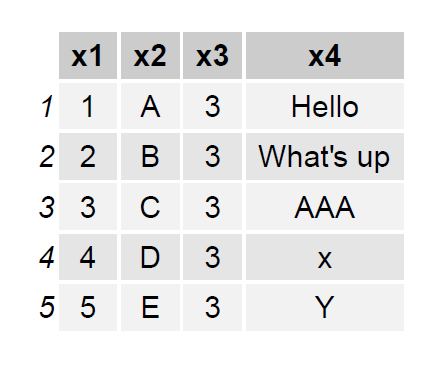# Export Nicely-Formatted Data Frame in R (2 Examples)

In this R tutorial you’ll learn how to return a nicely-formatted data frame as PDF or txt file.

The article consists of this content:

Let’s do this!

## Creation of Example Data

As first step, let’s create some example data in R:

```data <- data.frame(x1 = 1:5, # Example data x2 = LETTERS[1:5], x3 = 3, x4 = c("Hello", "What's up", "AAA", "x", "Y")) data # Return data to console # x1 x2 x3 x4 # 1 1 A 3 Hello # 2 2 B 3 What's up # 3 3 C 3 AAA # 4 4 D 3 x # 5 5 E 3 Y```

The previous output of the RStudio console shows the structure of our example data: It’s a data frame containing five rows and four columns.

## Example 1: Print Data Frame as PDF-File Using gridExtra Package

In Example 1, I’ll illustrate how to return a data set as PDF file using the gridExtra package. If we want to use the functions of the gridExtra package, we first need to install and load gridExtra:

```install.packages("gridExtra") # Install & load gridExtra library("gridExtra")```

Now, we can save our example data in a publication ready design by using the grid.table function as shown below:

```pdf("data_gridExtra.pdf") # Export PDF grid.table(data) dev.off()```Figure 1 shows the output of the previous R syntax – A pretty data matrix printed to a PDF file.

## Example 2: Print Data Frame as txt-File Using stargazer Package

This Example illustrates how to create a txt-file containing a nice looking data table. For this example, we are using the stargazer package:

```install.packages("stargazer") # Install & load gridExtra library("stargazer")```

Now, we can use the stargazer function to return our example data as txt:

```stargazer(data, # Export txt summary = FALSE, type = "text", out = "data_stargazer_txt.txt")```Figure 2 illustrates how our txt file looks like.

Note that you can use the stargazer function to export many different file-types such as LaTeX code or HTML/CSS code. You simply need to change the type argument within the stargazer command accordingly.

## Video, Further Resources & Summary

Some time ago I have released a video on my YouTube channel, which illustrates the R programming syntax of this post. You can find the video below.

Also, you could have a look at the related tutorials on my website:

This page showed how to print a pretty data table to paper in the R programming language. If you have any additional questions, don’t hesitate to let me know in the comments section below.